# Show classic screening curve analysis for generation investment¶

Compute the long-term equilibrium power plant investment for a given load duration curve (1000-1000z for z \in [0,1]) and a given set of generator investment options.

Available as a Jupyter notebook at http://www.pypsa.org/examples/generation-investment-screening-curve.ipynb.

In :
import pypsa
import numpy as np
import pandas as pd
%matplotlib inline

In :
#Generator marginal (m) and capital (c) costs in EUR/MWh - numbers chosen for simple answer
generators = {"coal" : {"m" : 2, "c" : 15},
"gas" : {"m" : 12, "c": 10},
"load-shedding" : {"m" : 1012, "c" : 0}}

In :
#Screening curve intersections at 0.01 and 0.5
x = np.linspace(0,1,101)
df = pd.DataFrame({key : pd.Series(item["c"] + x*item["m"],x) for key,item in generators.items()})
df.plot(ylim=[0,50],title="Screening Curve")

Out:
<matplotlib.axes._subplots.AxesSubplot at 0x7f9a9d17f390>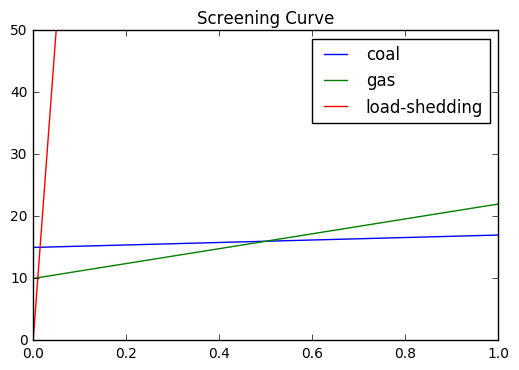In :
n = pypsa.Network()

num_snapshots = 1001

snapshots = np.linspace(0,1,num_snapshots)

n.set_snapshots(snapshots)

n.snapshot_weightings = n.snapshot_weightings/num_snapshots

p_set=1000-1000*snapshots)

for gen in generators:
p_nom_extendable=True,
marginal_cost=float(generators[gen]["m"]),
capital_cost=float(generators[gen]["c"]))

In :
n.loads_t.p_set.plot(title="Load Duration Curve")

Out:
<matplotlib.axes._subplots.AxesSubplot at 0x7f9ad01871d0>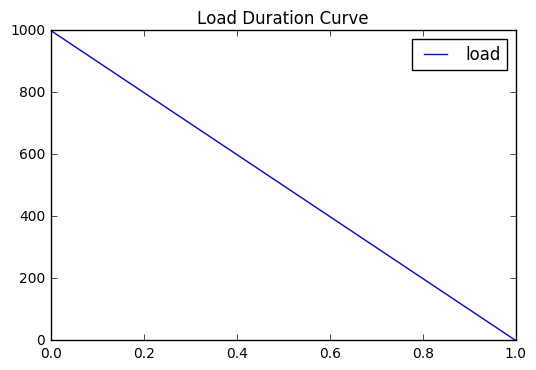In :
n.lopf(solver_name="cbc")

INFO:pypsa.pf:Slack bus for sub-network 0 is bus
INFO:pypsa.opf:Performed preliminary steps
INFO:pypsa.opf:Building pyomo model using angles formulation
INFO:pypsa.opf:Solving model using cbc
INFO:pypsa.opf:Optimization successful

Out:
('ok', 'optimal')
In :
print(n.objective)

14706.194

In :
#capacity set by total electricity required
#NB: no load shedding since all prices < 1e4
n.generators.p_nom_opt.round(2)

Out:
gas              490
coal             500
Name: p_nom_opt, dtype: int64
In :
n.buses_t.marginal_price.plot(title="Price Duration Curve")

Out:
<matplotlib.axes._subplots.AxesSubplot at 0x7f9a98d1f978>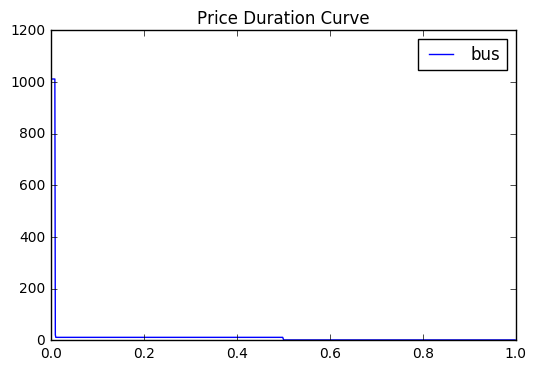In :
#The prices correspond either to VOLL (1012) for first 0.01 or the marginal costs (12 for 0.49 and 2 for 0.5)

#EXCEPT for (infinitesimally small) points at the screening curve intersections, which
#correspond to changing the load duration near the intersection, so that capacity changes
#This explains 7 = (12+10 - 15) (replacing coal with gas) and 22 = (12+10) (replacing load-shedding with gas)

#I have no idea what is causing \l = 0; it should be 2.

n.buses_t.marginal_price.round(2).sum(axis=1).value_counts()

Out:
2.0       499
12.0      489
1012.0     10
0.0         1
7.0         1
22.0        1
dtype: int64
In :
n.generators_t.p.plot(ylim=[0,600],title="Generation Dispatch")

Out:
<matplotlib.axes._subplots.AxesSubplot at 0x7f9a9d123400>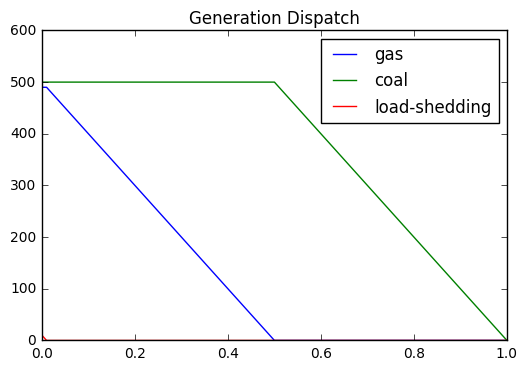In :
#Demonstrate zero-profit condition
print("Total costs:")
print(n.generators.p_nom_opt*n.generators.capital_cost + n.generators_t.p.multiply(n.snapshot_weightings,axis=0).sum()*n.generators.marginal_cost)

print("\nTotal revenue:")
print(n.generators_t.p.multiply(n.snapshot_weightings,axis=0).multiply(n.buses_t.marginal_price["bus"],axis=0).sum())

Total costs:
gas              6400.839161
coal             8249.750250
dtype: float64

Total revenue:
gas              6400.839108
coal             8249.750198
dtype: float64


# Without expansion optimisation¶

Take the capacities from the above long-term equilibrium, then disallow expansion.

Show that the resulting market prices are identical.

This holds in this example, but does NOT necessarily hold and breaks down in some circumstances (for example, when there is a lot of storage and inter-temporal shifting).

In :
n.generators.p_nom_extendable = False
n.generators.p_nom = n.generators.p_nom_opt

In :
n.lopf(solver_name='glpk')

INFO:pypsa.pf:Slack bus for sub-network 0 is bus
INFO:pypsa.opf:Performed preliminary steps
INFO:pypsa.opf:Building pyomo model using angles formulation
INFO:pypsa.opf:Solving model using glpk
INFO:pypsa.opf:Optimization successful

Out:
('ok', 'optimal')
In :
n.buses_t.marginal_price.plot(title="Price Duration Curve")

Out:
<matplotlib.axes._subplots.AxesSubplot at 0x7f9a98c58e10>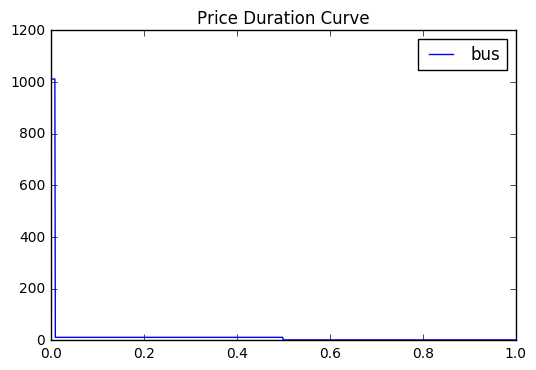In :
n.buses_t.marginal_price.sum(axis=1).value_counts()

Out:
2.0       500
12.0      490
1012.0     10
0.0         1
dtype: int64
In :
#Demonstrate zero-profit condition

#Differences are due to singular times, see above, not a problem

print("Total costs:")
print(n.generators.p_nom*n.generators.capital_cost + n.generators_t.p.multiply(n.snapshot_weightings,axis=0).sum()*n.generators.marginal_cost)

print("Total revenue:")
print(n.generators_t.p.multiply(n.snapshot_weightings,axis=0).multiply(n.buses_t.marginal_price["bus"],axis=0).sum())

Total costs:
gas              6400.839161
coal             8249.750250